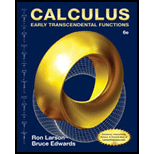Chapter 2.2, Problem 65ECalculus: Early Transcendental Fun...

6th Edition
Ron Larson + 1 other
ISBN: 9781285774770

Solutions

Chapter
SectionCalculus: Early Transcendental Fun...

6th Edition
Ron Larson + 1 other
ISBN: 9781285774770
Textbook Problem

Estimating a Limit Consider the function f ( x ) = ( 1 + x ) 1 / x ⋅ Estimate lim x → 0 ( 1 + x ) 1 / x by evaluating f at x-values near 0. Sketch the graph of f.

To determine

To Graph: Sketch the graph of the limit of the function f(x)=(1+x)1/x

Explanation

 X -0.1 -0.01 -0.001 0.001 0.01 0.1 f(x) 2.86797 2.73199 2.71964 2.71694 2.70481 2.59374

From the above table and graph, we could say that the value of the limit is 2.72. This is because f(x) approaches a unique value as X approaches 0 from either right or left.

Given: Function: f(x)=(1+x)1/x

Still sussing out bartleby?

Check out a sample textbook solution.

See a sample solution

The Solution to Your Study Problems

Bartleby provides explanations to thousands of textbook problems written by our experts, many with advanced degrees!

Get Started

In Exercises 516, evaluate the given quantity. log4116

Finite Mathematics and Applied Calculus (MindTap Course List)

True or False: converges.

Study Guide for Stewart's Multivariable Calculus, 8th

The graph of θ = 2 in polar coordinates is a: circle line spiral 3-leaved rose

Study Guide for Stewart's Single Variable Calculus: Early Transcendentals, 8th